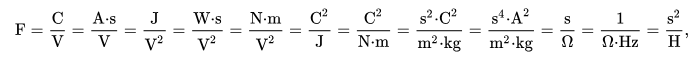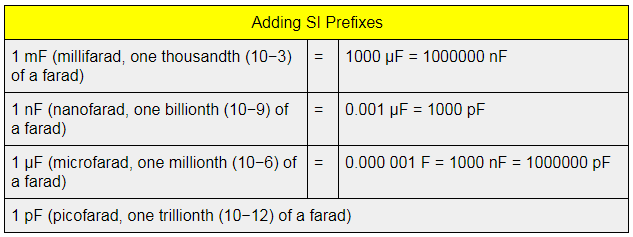# Unit of Capacitance

We can define capacitance as the ratio of the change in an electric charge in a system to the corresponding change in its electric potential. The unit of capacitance is provided in this article in a detailed manner so that learners can understand the concept easily.

Usually, there are two forms of capacitance namely self and mutual capacitance. We have covered the topic of capacitor and capacitance in our previous article which you can go through. However, in this page, we will learn about the measuring units.

## SI Unit of Capacitance

The SI unit of electrical capacitance is Farad which is represented by the symbol F. The unit is mainly named after English physicist Michael Faraday. Farad is also defined as the ability of an object or body to store an electrical charge. It is represented in terms of SI base units like s4⋅A2⋅m-2⋅kg-1. It can further be expressed as:Where,

• V is Volt
• A is Ampere
• J is Joule
• C is Coulomb
• N is Newton
• m is Metre
• W is Watt
• s is Second
• kg is Kilogram
• Hz is Hertz
• Ω is Ohm
• H is Henry

While the basic unit of capacitance is farad, but this unit is actually too big for any practical work. To make things easier, the capacitance is also measured in microfarads abbreviated as μF or mfd and picofarads abbreviated as pF.### Other Capacitance Units

There are various other units of capacitance. Some of the other units are provided below.

• Abfarad (abbreviated abF) which is an obsolete CGS unit of capacitance equal to 109 farads (1 gigafarad, GF).
• Another rarely used CGS unit is statfarad (abbreviated statF) and it is equivalent to the capacitance of a capacitor with a charge of 1 statcoulomb across a potential difference of 1 statvolt. In terms of farad, it is 1.1126 x 10-12 which is approximately 1.1126 picofarads.

### Summary

 Common symbols C SI unit Farad (F) In SI base units F = A2 s4 kg−1 m−2 Other units μF, nF, pF Dimension M−1L−2T4I2

Related Articles:

Q1

One Farad is defined as the capacitance for a particle with a one-coulomb charge and with the potential difference of one volt.

Q2

### What is the relationship between capacitance, charge, and potential difference?

The relationship between capacitance, charge, and potential difference is that they are linear. That is when the potential difference is halved, then the quantity of charge stored in the capacitor is halved.

Q3

### What type of energy is stored in a capacitor?

Potential energy is stored in the capacitor. This is because the capacitor stores the charge between the plates and these charges are stationary. Therefore, the energy stored is potential energy.

Q4

### Why is a capacitor considered to be a passive device?

Capacitor is considered to be a passive device because it consumes power.

Q5

### Name a material with high capacitance.

Metals have high capacitance as they have high dielectric constant value.Test your Knowledge on unit of capacitance